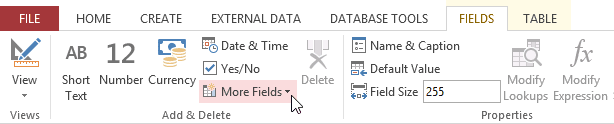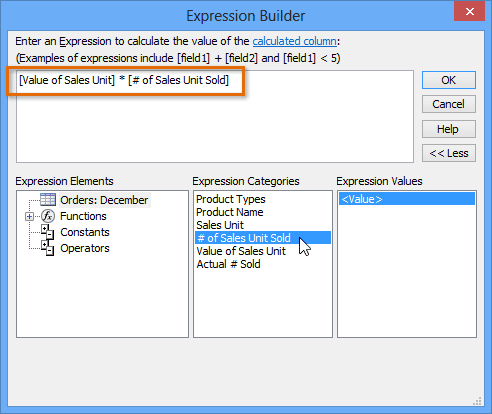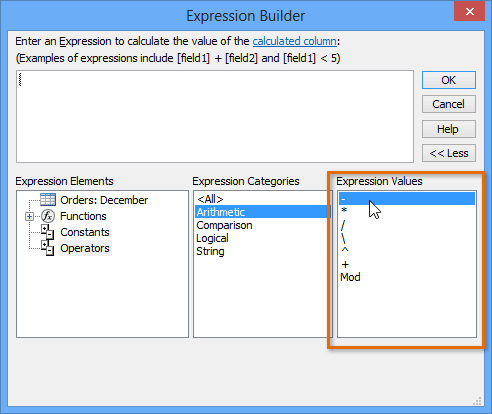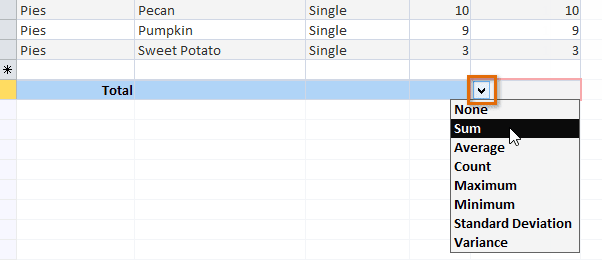# Creating Calculated Fields and Totals Rows

Calculated fields and totals rows let you perform calculations with the data in your tables. Calculated fields perform calculations using data within one record, while totals rows perform a calculation on an entire field of data.

Calculated fields

When you create a calculated field, you are adding a new field in which every row contains a calculation involving other numerical fields in that row. To do this, you must enter a mathematical expression, which is made up of field names in your table and mathematical symbols. You don't need to know too much about math or expression building to create a useful calculated field. In fact, you can write robust expressions using only grade-school math. For instance, you could:

Use + to find the sum of the contents of two fields or to add a constant value (such as + 2 or + 5) to a field.

Use * to multiply the contents of two fields or to multiply fields by a constant value.

Use - to subtract one field from other or to subtract a constant value from a field.

In our example, we will use a table containing the orders from one month. The table contains items listed by sales unit - single, half-dozen, dozen. One column lets us know the number sold of each sales unit. Another lets us know the actual numerical value of each of these units. For instance, in the top row you can see that two dozen fudge brownies have been sold and that one dozen equals 12 brownies.

To find the total number of brownies that have been sold, we'll have to multiply the number of units sold by the numerical value of that unit—here, 2*12, which equals 24. This was a simple problem, but performing that calculation for each row of the table would be tedious and time consuming. Instead, we can create a calculated field that shows the product of these two fields multiplied together on every row.

To create a calculated field

1. Select the Fields tab, locate the Add & Delete group, and click the More Fields drop-down command.2. Hover your mouse over Calculated Field and select the desired data type. We want our calculation to be a number, so we'll select Number.3. Build your expression. To select fields to include in your expression, double-click the field in the Expression Categories box. Remember to include mathematical operators like the + or - signs. Because we want to multiply our two fields, we'll put the multiplication symbol (*) between them.4. Click OK. The calculated field will be added to your table. If you want, you can now sort or filter it.5. For more examples of mathematical expressions that can be used to create calculated fields, review the Arithmetic Expressions in the Expression Builder dialog box.Totals rows

The totals row adds up an entire column of numbers, just like in a ledger or on a receipt. The resulting sum appears in a special row at the bottom of your table.

For our example, we'll add a totals row to our calculated field. This will show us the total number of items sold.

To create a totals row

1. From the Home tab, locate the Records group, then click the Totals command.2. Scroll down to the last row of your table.

3. Locate the desired field for the totals row, then select the second empty cell below the last record for that field. When a drop-down arrow appears, click it.4. Select the function you want to perform on the field data. In our example, we'll choose Sum to add all of the values in the calculated field.5. The totals row will appear.